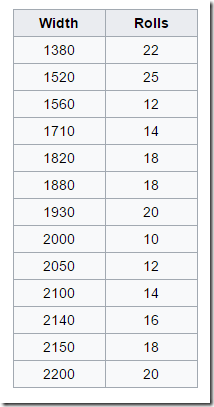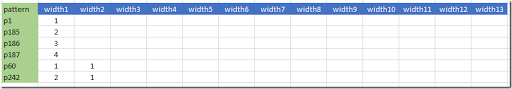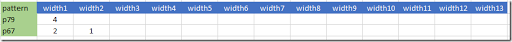## Friday, March 24, 2017

### Cutting Stock Problem: Generating all patterns by MIP Solution Pool

In (1) a small cutting stock problem is described. We need to cut up raw material rolls of width 5600 mm into the following pieces:In this post I want to explore how we can use the solution pool technology in Cplex and Gurobi to do some of the heavy lifting for us.

##### Generating all possible patterns

From (1):

There are 308 possible patterns for this small instance.

Enumerating those patterns can be done by writing your own algorithm in your favorite programming language. Alternatively we can develop a small MIP model and let the solution pool do the job for us. See (2) for another example of this.

A minimalistic model is:

 \bbox[lightcyan,10px,border:3px solid darkblue]{\begin{align}\min\>&0\\ &L \le \sum_j w_j y_j \le R\\ &y_j \in \{0,1,2,\dots,q_j\}\end{align}}

were $$w_j,q_j$$ are the width and rolls from the demand table above, $$R=5600$$, and $$L=\displaystyle\min_j w_j$$.

There is a dummy objective: we are just looking for feasible solutions. The lower limit of $$L$$ is there to exclude a pattern with all $$y_j=0$$.

The solution pool will be able to find all feasible integer solutions for this model very fast.

When we solve this with GAMS/Cplex we see:

 Total (root+branch&cut) =    0.13 sec. (4.46 ticks)Dumping 308 solutions from the solution pool...

This model is probably shorter than anything we can program in a standard programming language.

Now we have a collection of all patterns $$a_{i,j}$$ formed by collecting all $$y_j$$ we found. Note that we enumerated literally all possible patterns including things like:E.g. pattern 1 has only one item: a single roll with a width of 1380 mm.

##### Solving the cutting stock problem: minimize waste

The subsequent cutting stock problem can be formulated as (1):

 \bbox[lightcyan,10px,border:3px solid darkblue]{\begin{align}\min\>&\sum_i c_i x_i\\ &\sum_i a_{i,j} x_i \ge q_j\>\>\forall j\\ &x_i \in \{0,1,2,\dots\}\end{align}}

The cost coefficients $$c_i$$ correspond to cost or waste of each pattern $$i$$, i.e. $$c_i = R-\displaystyle\sum_j w_j a_{i,j}$$.

Actually this model is not completely correct. Some of the patterns have a zero waste, E.g.:

These two patterns have a total width of $$1520+1880+2200=1520+1930+2150=5600$$. As a result the model can use any amount of these patterns without cost. Indeed I saw a strange solution:

 ----    109 VARIABLE x.L  p18     14,    p38     10,    p40      4,   p68  99999,    p70     16,    p81  99999,    p142     4,    p159     8p187     2

Indeed these two "zero waste" patterns have bad values.

We can correct this easily by changing the model to:

 \bbox[lightcyan,10px,border:3px solid darkblue]{\begin{align}\min\>&\mathit{waste} = \sum_i c_i x_i\\ &\sum_i a_{i,j} x_i = q_j\>\>\forall j\\ &x_i \in \{0,1,2,\dots\}\end{align}}

i.e. use an equality constraint. Now we get a proper solution:

 ----    109 VARIABLE x.L  p18   6,    p30   1,    p38   8,    p40   7,    p68   5,    p70  15,    p75   1,    p81   4,    p121  4,    p142  7p156  5,    p228  6,    p277  1,    p282  3 ----    114 PARAMETER n                    =           73  total amount of stock used ----    118 PARAMETER waste_percent        =        0.401  waste as percentage of material

Here we calculated:

 \begin{align}&n=\sum_i x^*_i\\ &\mathit{waste}\% = 100 \frac{\mathit{waste}^*}{n\cdot R}\end{align}

The numbers for total used raw material and percentage waste are the same as mentioned in (1).

##### Multiple solutions

The optimal combination of patterns to cut is not unique. One interesting subset of solutions is the one with a minimum number of different patterns. One way to find this solution is by solving a subsequent model, with the optimal amount of raw as constraint:

 \bbox[lightcyan,10px,border:3px solid darkblue]{\begin{align}\min\>&\mathit{numPatterns} = \sum_i \delta_i\\ &\sum_i a_{i,j} x_i = q_j\\ &\sum_i x_i = n\\ &x_i \le n \delta_i\\ &\delta_i \in \{0,1\}\\ &x_i \in \{0,1,2,\dots\}\end{align}}

This gives indeed what we want: 10 different patterns:

 ----    139 VARIABLE numPatterns.L         =           10  ----    139 VARIABLE x.L  p40   7,    p43   3,    p68   8,    p70  11,    p81   6,    p115 10,    p123  2,    p142 12,    p228 12,    p280  2

What about generating all possible optimal solutions for this? In (1) it is stated there are 19 solutions with 10 different patterns. Again we can use the solution pool for this. We see:

 Total (root+branch&cut) =    3.22 sec. (794.99 ticks)Dumping 19 solutions from the solution pool...
##### Minimize number of raw material rolls

Instead of minimizing waste we can also just minimize the number of raw material rolls. This corresponds to $$c_i=1$$. Solving the cutting stock model with this objective gives the same result.

In this case we can actually make the model much smaller by only considering “full” patterns. That is, only consider patterns that have no extra space left for another item from our demand data set.

From our original example:we only want to keep rows that are non-dominated (the pattern numbers are reordered, so they don’t correspond):The model to generate these can be written as:

 \bbox[lightcyan,10px,border:3px solid darkblue]{\begin{align}\min\>&0\\ &r = R-\sum_j w_j y_j\\ &y_j \ge (1-\delta_j) q_j&\forall j\\ &r \le w_j \delta_j + R(1- \delta_j)&\forall j\\ &r \ge 0\\ &\delta_j \in \{0,1\}\\ &y_j \in \{0,1,2,\dots,q_j\}\end{align}}

The binary variable $$\delta_j\in\{0,1\}$$ is forced to assume the value of one when we still have a demand left for another item $$j$$. We require that the remaining space available is smaller than the space needed to place any remaining demand.

Note that the second constraint $$y_j \ge (1-\delta_j) q_j$$ implements the implication:

 $y_j while the third constraint $$r \le w_j \delta_j + R(1- \delta_j)$$ yields:  \[0 \le r \le \min_{j|\delta_j=1}w_j$

and so forcing the remaining space $$r$$ to be smaller than any demanded item we still can assign. When we solve this model with the solution pool we find just 213 different patterns (compared to 308 before). The subsequent cutting stock model can look like:

 \bbox[lightcyan,10px,border:3px solid darkblue]{\begin{align}\min\>&n=\sum_i x_i\\ &\sum_i a_{i,j} x_i \ge q_j\>\>\forall j\\ &x_i \in \{0,1,2,\dots\}\end{align}}

We find the same $$n=73$$ as before:

 ----    199 PARAMETER n                    =           73  total amount of stock used ----    199 VARIABLE x.L  p21  16,    p26   6,    p48   3,    p94   8,    p102  3,    p106 12,    p117  2,    p124  7,    p131  1,    p135  1p188 12,    p208  2,    p228  1,    p280  2
##### Conclusion

The solution pool technique can be a useful tool for smaller instances of cutting stock problems where we want to enumerate patterns or optimal solutions. Of course for large problems these enumeration steps are not practical.

##### References
1. Cutting stock problem, https://en.wikipedia.org/wiki/Cutting_stock_problem
2. Employee Scheduling III: generating all shifts using the Cplex solution pool, http://yetanothermathprogrammingconsultant.blogspot.com/2017/01/employee-scheduling-iii-generating-all.html